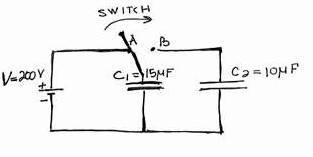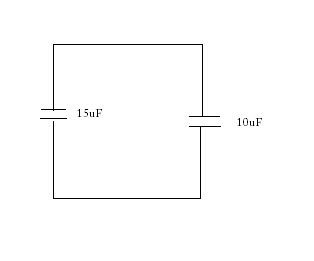# A battery, two capacitors, and a switch

## Homework Statement

If the switch is flipped from position A to position B, what's the charge on capacitor C1 and C2?Q=CV

## The Attempt at a Solution

I know that the capacitor C1 gains potential difference of 200V (= potential difference of a battery) and has charge Q = C1*200, but I'm not sure what happens once the flip is switched. My assumption is that it must discharge because it is no longer connected to the battery, but does it discharges until Q = 0 or until the charge on C2 = charge on C1. Could somebody please explain to me what exactly happens once the switch is in position B
Thank you!

Charge moves down the potential hill. (conventional)

mjsd
Homework Helper
from conservation of charge: amount of charge stored when in position A must be same as in position B, the only different is that now you have two capacitors to share the storage.. now, assuming that the second capacitor is initially uncharged, then the total charge in the two-capacitor system must be the same as before... due to how the circuit is set up the voltage across the two capacitor must also equal...

from conservation of charge: amount of charge stored when in position A must be same as in position B, the only different is that now you have two capacitors to share the storage.. now, assuming that the second capacitor is initially uncharged, then the total charge in the two-capacitor system must be the same as before... due to how the circuit is set up the voltage across the two capacitor must also equal...

I got it. Thank you very much. I knew about the voltage, but I forgot to consider charge conservation. Thanks!

I'm trying to solve a similar problem and I understand everything except the following part:

Why are C1 & C2 in parallel after flipping the switch to B?

Doesn't the circuit just look like this after the switch goes to B (ignoring the part of the circuit where they connect to the battery because that is not functional now):So aren't they really in serial?